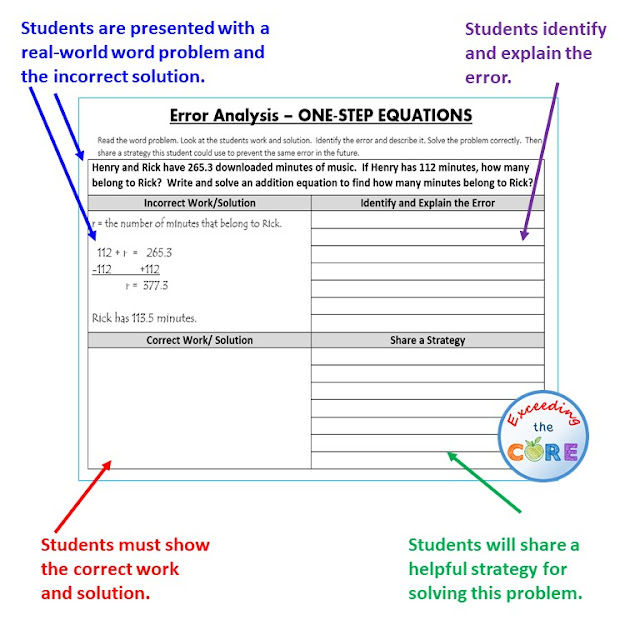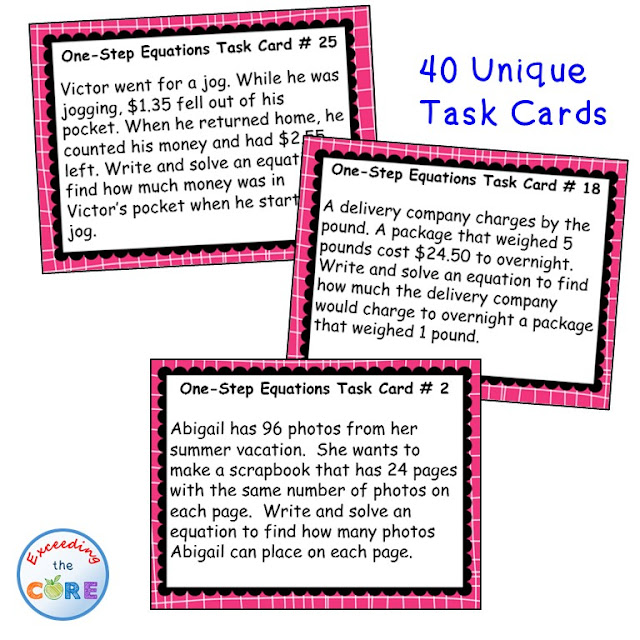### Write and Solve One-Step Equations

Introduce your students to writing and solving one-step equations with a variety of engaging activities.  When teaching students how to solve equations, you want them to understand that they need to perform the inverse (opposite) operation of the operation that is given.  This will allow them to isolate the variable.

I have created and used all of the resources shown below with my middle school students.  Each of these activities gives my students a variety of opportunities to master writing and solving one-step equations.

This One-Step Equations UNIT RESOURCE BUNDLE includes 40 task cards, 10 error analysis activities and 10 problem solving graphic organizers, 4 homework practice worksheets, 1 maze worksheet, 1 riddle worksheet, 1 coloring page activity (over 125 skills practice and real-world word problems).

Topics included:

💥 Solve & Write Addition Equations

💥 Solve & Write Subtraction Equations

💥 Solve & Write Multiplication Equations

💥 Solve & Write Division Equations

The following math activities are perfect for warm-ups, cooperative learning, spiral review, math centers, assessment prep and homework.  There is a printed and digital version for each activity.

This unit resource bundle includes:

Each page has a real-world word problem that is solved incorrectly. Students have to identify the error, provide the correct solution and share a helpful strategy for solving the problem. Print or use the digital version of this activity.One-Step Equations Error Analysis Activity

Each worksheet presents students with a real-word problem. Students must then organize the information using a problem-solving graphic organizer. Students are prompted to identify the important information in the word problem, solve, justify their work and explain their solution.

Each task card has a real-world word problem. Students can work on these cards individually, with partners or in groups.One-Step Equations Word Problem Task Cards

Each worksheet includes two sections, Skills Practice and Problems Solving. The SKILLS PRACTICE section of each worksheet includes 6 computational problems to help students sharpen their skills. The WORD PROBLEM section of each worksheet allows students to apply their understanding of the topic to solve 4 real-world problems.One-Step Equations Skills Practice and Problem Solving Activity

A maze, riddle and coloring page is also included. All 3 of these activities allow students to practice this skill while incorporating fun into the classroom! Print or use the digital version of this activity.One-Step Equations Riddle, Maze and Coloring Page